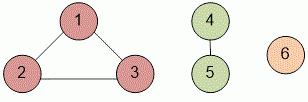Problems

# Connected components

The undirected unweighted graph is given. Find the number of its connected components.

#### Input

The first line contains the number of vertices n (n100) in a graph. In each of the next n rows n numbers are given - the adjacency matrix of the graph: in the i-th row of the j-th place there is a 1 if the vertices i and j are connected by an edge, and 0 otherwise. The main diagonal of the matrix contains zeroes. The matrix is symmetric with respect to the main diagonal.

#### Output

Print the number of connected components in a graph.Time limit 1 second
Memory limit 128 MiB
Input example #1
6
0 1 1 0 0 0
1 0 1 0 0 0
1 1 0 0 0 0
0 0 0 0 1 0
0 0 0 1 0 0
0 0 0 0 0 0

Output example #1
3

Source 2011 Summer school, Sevastopol, August 8, Day 1, League 1